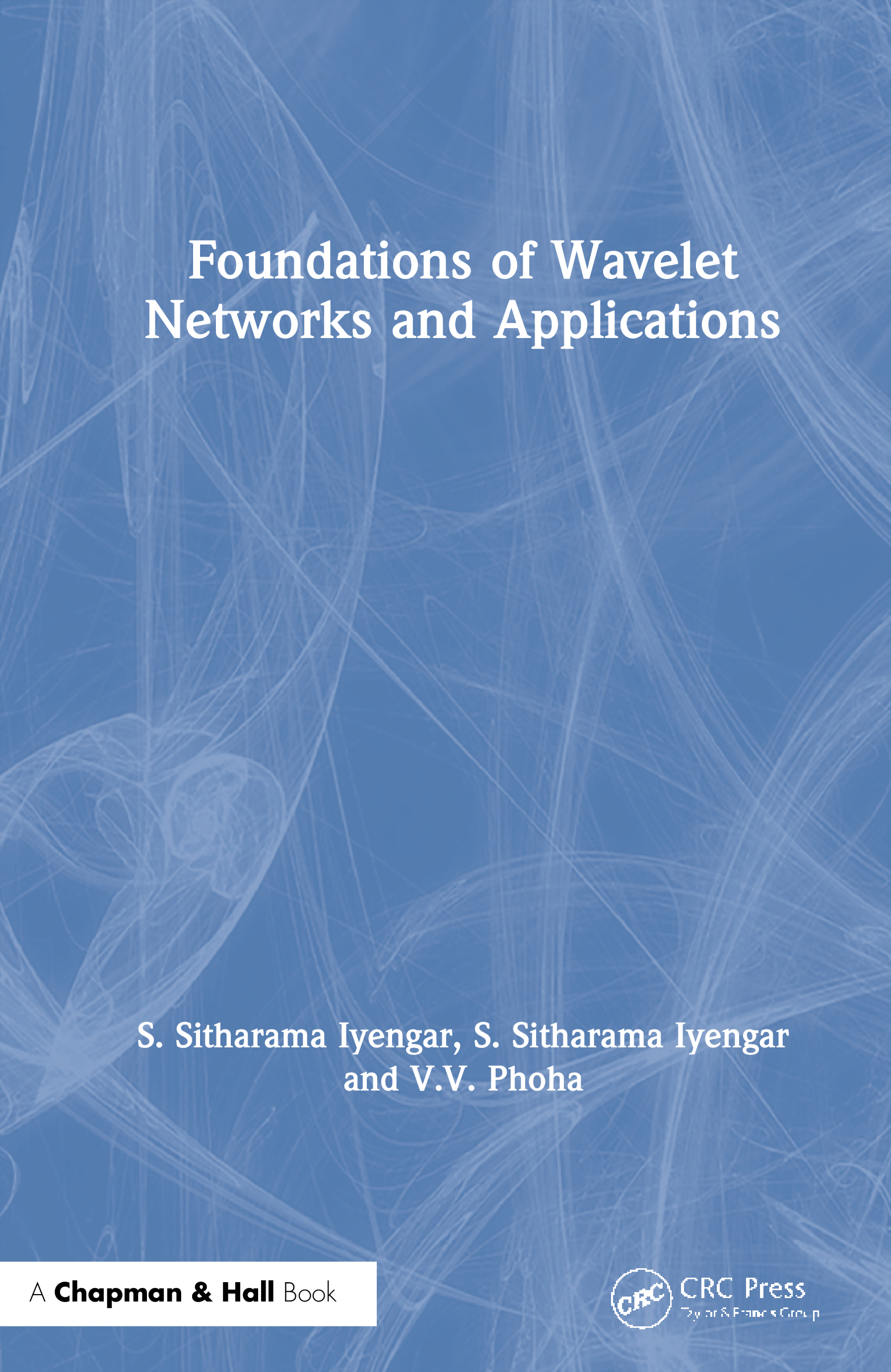# Foundations of Wavelet Networks and Applications

## 1st Edition

Chapman and Hall/CRC

288 pages | 66 B/W Illus.

##### Purchasing Options:\$ = USD
Hardback: 9781584882749
pub: 2002-06-27
SAVE ~\$28.00
\$140.00
\$112.00
x
eBook (VitalSource) : 9781315273679
pub: 2018-10-08
from \$70.00

FREE Standard Shipping!

### Description

Traditionally, neural networks and wavelet theory have been two separate disciplines, taught separately and practiced separately. In recent years the offspring of wavelet theory and neural networks-wavelet networks-have emerged and grown vigorously both in research and applications. Yet the material needed to learn or teach wavelet networks has remained scattered in various research monographs.

Foundations of Wavelet Networks and Applications unites these two fields in a comprehensive, integrated presentation of wavelets and neural networks. It begins by building a foundation, including the necessary mathematics. A transitional chapter on recurrent learning then leads to an in-depth look at wavelet networks in practice, examining important applications that include using wavelets as stock market trading advisors, as classifiers in electroencephalographic drug detection, and as predictors of chaotic time series. The final chapter explores concept learning and approximation by wavelet networks.

The potential of wavelet networks in engineering, economics, and social science applications is rich and still growing. Foundations of Wavelet Networks and Applications prepares and inspires its readers not only to help ensure that potential is achieved, but also to open new frontiers in research and applications.

### Reviews

"This book reviews both the theory of some kinds of wavelet networks and a number of applications … . The book is self-contained, as it contains both some mathematical preliminaries and a review of fundamentals about wavelets as well as neural networks. Moreover, at the end of each chapter it contains a number of exercises useful to help the reader to verify the degree of his/her understanding … . The book is highly recommended to all those looking for new methods in neural networks devoted to signal analysis."

- Mathematical Reviews, Issue 2005d

PART A

MATHEMATICAL PRELIMINARIES

Sets

Functions

Sequences and Series

Complex Numbers

Linear Spaces

Matrices

Hilbert Spaces

Topology

Measure and Integral

Fourier Series

Exercises

WAVELETS

Introduction

Dilation and Translation

Inner Product

Haar Wavelet

Multiresolution Analysis

Continuous Wavelet Transform

Discrete Wavelet Transform

Fourier Transform

Discrete Fourier Transform

Discrete Fourier Transform of Finite Sequences

Convolution

Exercises

NEURAL NETWORKS

Introduction

Multilayer Perceptrons

Hebbian Learning

Competitive and Kohonen Networks

Recurrent Neural Networks

WAVELET NETWORKS

Introduction

What Are Wavelet Networks

Theory of Wavelet Networks

Wavelet Network Structure

Multidimensional Wavelets

Learning in Wavelet Networks

Initialization of Wavelet Networks

Properties of Wavelet Networks

Scaling at Higher Dimensions

Exercises

PART B

RECURRENT LEARNING

Introduction

Recurrent Neural Networks

Recurrent Wavenets

Numerical Experiments

Concluding Remarks

Exercises

SEPARATING ORDER FROM DISORDER

Order Within Disorder

Comparison Results

Conclusions

Exercises

RADIAL WAVELET NEURAL NETWORKS

Introduction

Data Description and Preparation

Classification Systems

Results

Conclusions

Exercises

PREDICTING CHAOTIC TIME SERIES

Introduction

Nonlinear Prediction

Wavelet Networks

Short-Term Prediction

Parameter-Varying Systems

Long-Term Prediction

Conclusions

Acknowledgements

Appendix

Exercises

CONCEPT LEARNING

An Overview

An Illustrative Example of Learning

Introduction

Preliminaries

Learning Algorithms

Summary

Exercises

BIBLIOGRAPHY

INDEX

### Subject Categories

##### BISAC Subject Codes/Headings:
COM051240
COMPUTERS / Software Development & Engineering / Systems Analysis & Design
COM059000
COMPUTERS / Computer Engineering
MAT003000
MATHEMATICS / Applied